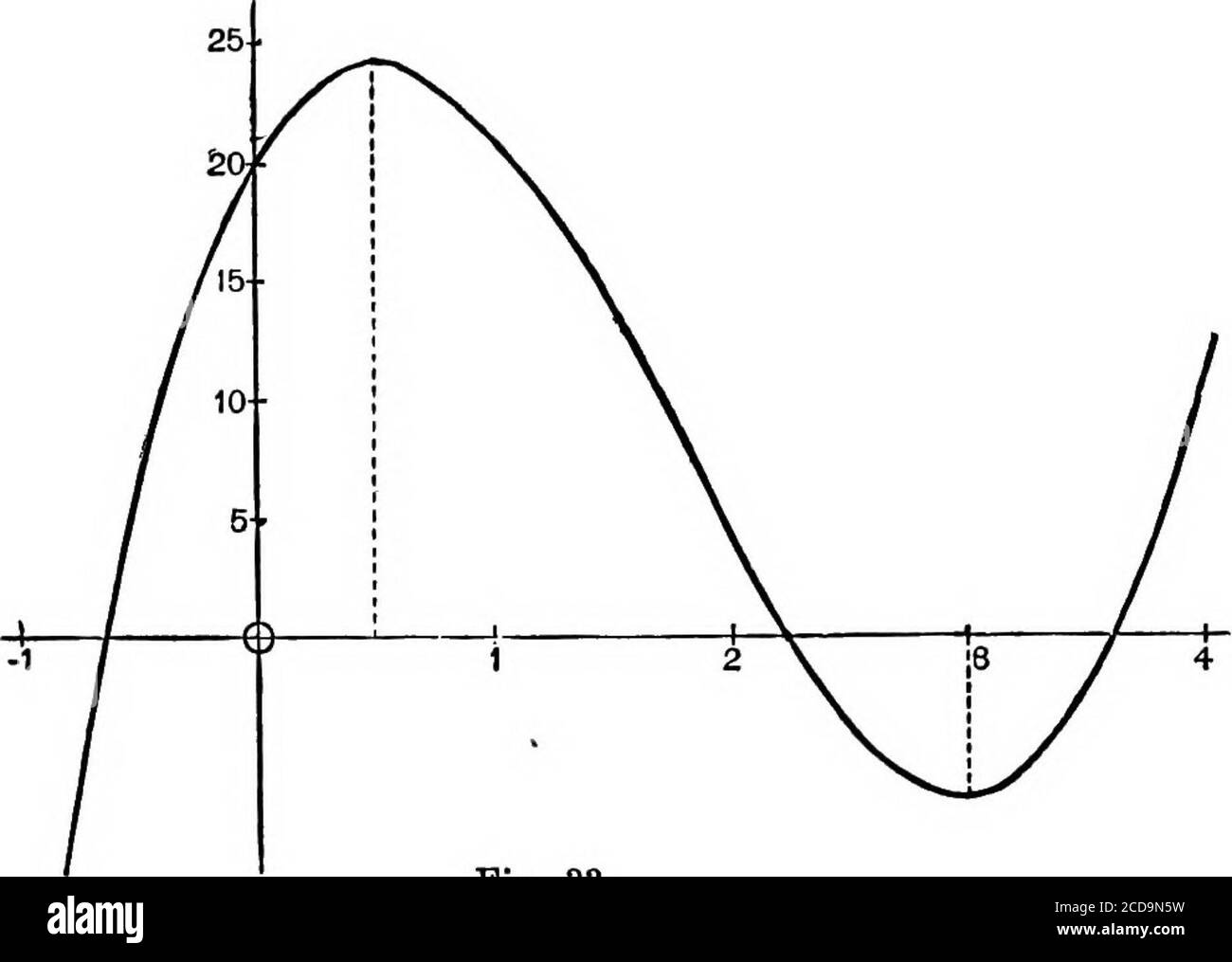# . An elementary course of infinitesimal calculus . s derivative ^(x) are both of them continuous (andfinite) for all finite values of x. Hence at least one real rootof the equation (x) = 0 (1) 48-49] APPLICATIONS OF THE DERIVED FUNCTION. 105 will lie between any two real roots of «^(^) = 0 (2). This result, which is known as RoUes Theorem, is importantin the Theory of Equations. It is an immediate consequencethat at most one real root of (2) lies between any twoconsecutive roots of (1). That is, the roots of (1) separatethose of (2). JSx. 1. If &lt;^ (x) = 4a!» - 2la? + ISa; + 20,we have &lt;^## Image details

Contributor:

Reading Room 2020 / Alamy Stock Photo

Image ID:

2CD9N5W

File size:

Releases:

Model - no | Property - noDo I need a release?

Dimensions:

1874 x 1334 px | 31.7 x 22.6 cm | 12.5 x 8.9 inches | 150dpi

This image is a public domain image, which means either that copyright has expired in the image or the copyright holder has waived their copyright. Alamy charges you a fee for access to the high resolution copy of the image.

This image could have imperfections as it’s either historical or reportage.

. An elementary course of infinitesimal calculus . s derivative ^(x) are both of them continuous (andfinite) for all finite values of x. Hence at least one real rootof the equation (x) = 0 (1) 48-49] APPLICATIONS OF THE DERIVED FUNCTION. 105 will lie between any two real roots of «^(^) = 0 (2). This result, which is known as RoUes Theorem, is importantin the Theory of Equations. It is an immediate consequencethat at most one real root of (2) lies between any twoconsecutive roots of (1). That is, the roots of (1) separatethose of (2). JSx. 1. If &lt;^ (x) = 4a!» - 2la? + ISa; + 20,we have &lt;^ (x) = 120? - 42a! + 18 = 6 (2a; - 1) (a; - 3). Hence the real roots of &lt;^ (x) = 0, if any, will lie in the intervalsbetween — oo and J, ^ and 3, 3 and + oo , respectively. Now, for as = — 00 , J, 3, + 00,the signs of (x) are —, +, —, +, respectively, so that ij&gt; (x) must in fact vanish once (by Art. 10)in each of the above intervals. Hence there are three realroots. The figure shews the graph of (a!).. Fig. 33. If by continuous modification of the form of &lt;j){x), forexample by the addition or subtraction of a constant, tworoots are made to coalesce, the root of (x) = 0 which liesbetween must coalesce with them. Hence a double root of&lt;^(a!) = 0 is also a root of &lt;j)(x) = 0. 106 INFINITESIMAL CALCULUS. [CH. Ill More generally, an r-fold root of ^ (a;) = 0 being regardedas due to the coalescence of r distinct roots, the equation&lt;^ (x) = 0 will have r — 1 intent ening roots which coalesce. This suggests a method of ascertaining the multiple roots,if any, of a proposed algebraic equation. If a be an r-foldroot of &lt;^(a;), we have {x) = {x-arx{oo) (3). where % (x) is a rational integral function. Hence f {x) = {x- ay-^ [rx (x) + (x-a) x (ix:)} (4); i.e. (x — ay~^ will be a common factor of &lt;^(«) and ^{x).And it- is easily seen that (x — a)^^ will not be a commonfactor unless ^ {x) is divisible by (x — ay. Hence themultiple roots of ^ (x), if

Save up to 30% with our image packs